#### Methods, Associated functions, Converting Python to Rust, and MoreHealth vector created by pikisuperstar — www.freepik.com

### Introduction

Operators tell the compiler or interpreter to perform a specific mathematical, relational, or logical operation. Many programming languages make use of similar operator symbols.

We will go through the important arithmetic, relational, and logical operators available in Rust and we will compare them to Python.

We will learn the differences between methods and associated functions.

We also convert two simple Python codes to Rust codes to learn more about Rust programming.

Let’s get started!

Learning Rust by Converting Python to Rust

### Arithmetic Operators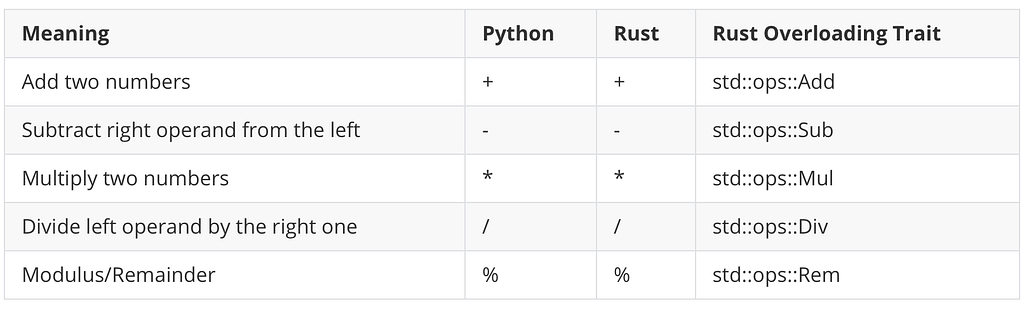Rust and Python Arithmetic Operators. Image by the author.

Python and Rust share the same arithmetic symbols as you see in the above table. Rust calls % as Remainder instead of the Modulus.

Output:

`a: 20, b: 20+1=21, c: 20-2=18, d: 20*3=60, e: 20/4=5, f: 20%3=2`

In Rust, you can’t use different data types in an operation. For example, if you try to subtract an unsigned integer from a signed integer, it will fail:

`// This will fail.fn main() {    let a = 8u8;    let b = 2i32;    println!("{}", a - b);}`

Rust uses the as keyword to cast between primitive types. Please read more about the cast in Rust here.

Output:

`6`

#### Exponent

Python uses the ** symbol for exponents:

Output:

`2^3 is  83^3 is  273^3.2 is  33.63473536961897`

Rust uses pow, powi, and powf depends on the type:

Output:

`2 ^ 3 in Rust: 2u8.pow(3) = 82 ^ 3 in Rust: 2i32.pow(3) is 83.0 ^ 3 in Rust: 3.0f32.powi(3) 273.0 ^ 3.2 in Rust: 3.0_f32.powf(3.2) is 33.63474a = 3, a ^ 3 in Rust: i32::pow(a,3) = 27b = 3.1, b ^ 3 in Rust: f64::powi(b, 3) = 29.791000000000004b = 3.1, b ^ PI in Rust: std::f64::consts::PI) = 34.96699308140392`

In Rust, you can annotate a number type like 2u8 or 2_u8. u8 is an unsigned 8-bit integer type and i32 is a signed integer type.

i32 and f32 have a group of built-in methods. All the integer types u8, u16, u32, u64, u128, i16,i32, i64 , i128, isize, and usize have the pow method.

`pub fn pow(self, exp: u32) -> i32`

The above definition tells you that using the pow method raises self to the power of exp (which is u32) and returns i32 (a signed integer).

The floating-point types, f32 and f64 have powi and powf methods.

powi raises a number to an integer power and powf raises a number to a floating-point power.

`pub fn powi(self, n: i32) -> f32pub fn powf(self, n: f32) -> f32`

#### Floor Division

In Python, we use // to find a floor division. For example 5//2=2.

Output:

`5 // 2 is  2-5 // 2 is  -3`

Output:

`2-3`

### Comparison Operators

Python and Rust share the same symbols for all the comparison operators.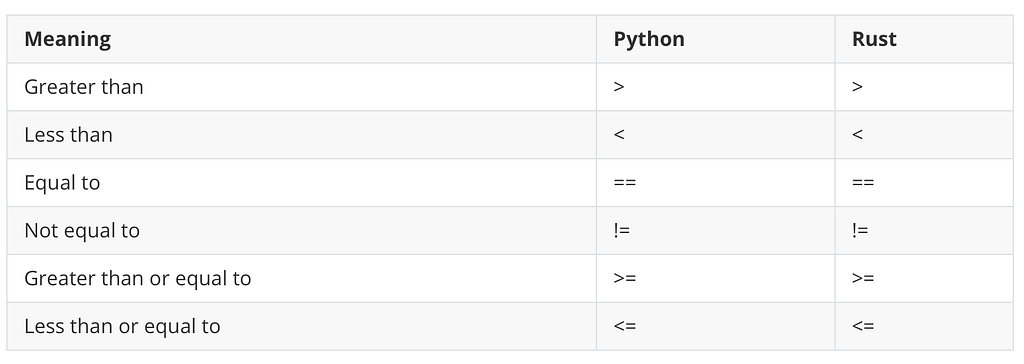Rust and Python Comparison Operators. Image by the author.

Output:

`    a: 7, b: 4,     c: 7 == 4 is false,     d: 7 != 4 is true,     e: 7<4 is false,     f: 7>4 is true,     g: 7<=7 is true,     h: 7>=7 is true`

### Logical Operators

Rust logical operator symbols are different from Python ones.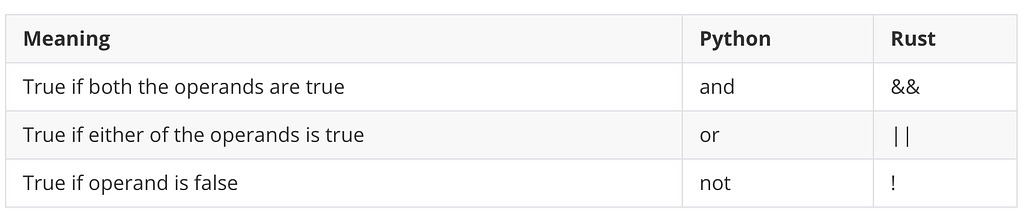Rust and Python Logical Operators. Image by the author.

Output:

`    a: true, b: false,     c: !true is false,     d: true && false is false,     e: true || false is true`

### Bitwise Operators

All the Rust and Python Bitwise operators share the same bitwise operator symbols except the bitwise NOT.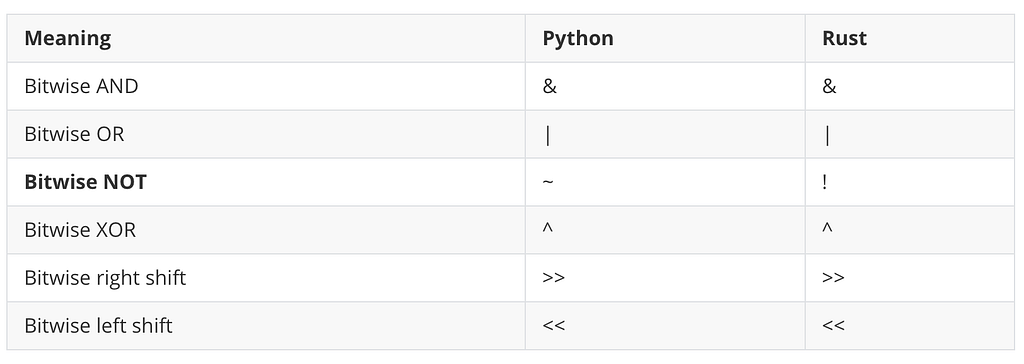Rust and Python Bitwise Operators. Image by the author.

Output:

`    a: 1, b: 2,     c: 1 & 2 is 0,     d: 1 | 2 is 3,     e: 1 ^ 2 is 3,    f: 1 << 2 is 4,    f2: 1 << 4 is 16,    g: 1 >> 2 is 0,    g2: 1 >> 2 is 1,    h: !1 = -2`

Bitwise negation !1 returns -2. Rust uses the two’s complement to find the bitwise negation for signed types. Rust’s signed integer types are called the signed two’s complement integer types.

You can use 1 << n to find out exponents of 2.

Output:

`2 ^ 3 = 82 ^ 4 = 162 ^ 5 = 32`

### Compound Assignment Operators

All the Rust and Python compound assignment operators have the same symbols except Rust doesn’t have the equivalence of power assignment **=, and floor division assignment //=.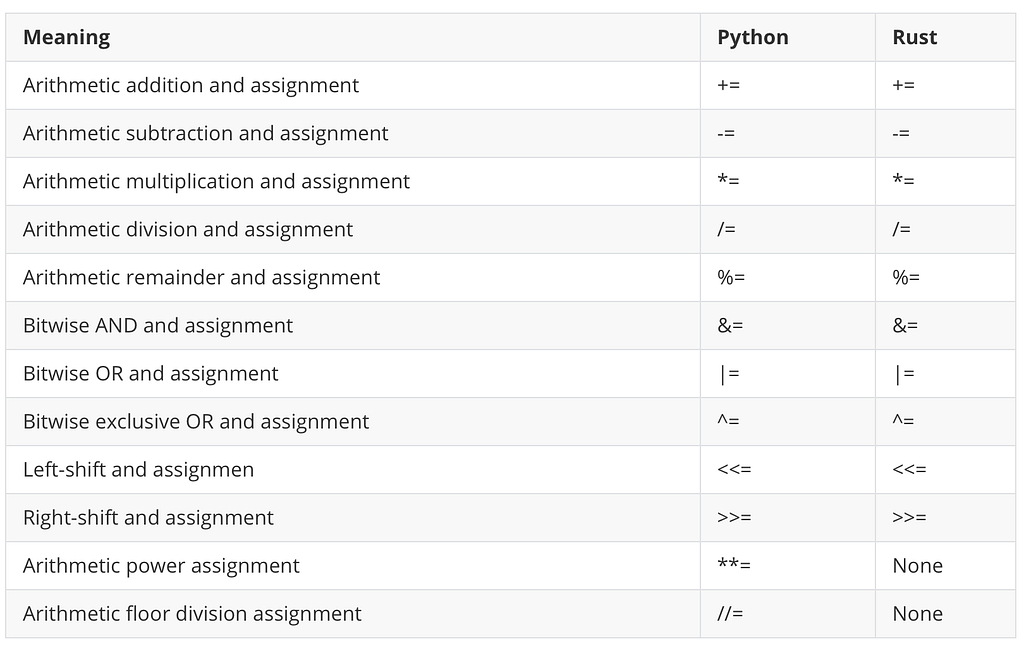Rust and Python Compound Assignment Operators

Output:

`a is 21: a += 5 is 72: a -= 2 is 53: a *= 5 is 254: a /= 2 is 125: a %= 5 is 26: a &= 2 is 27: a |= 5 is 78: a ^= 2 is 59: a <<= 1 is 1010: a >>= 2 is 2`

Operator overloading is to specify more than one definition for an operator in the same scope. Python and Rust provide operator overloading. You can find Rust overloadable operators in the standard library ops module.

Output:

`Point { x: 3, y: 3 }`

### XOR and Bitwise Operators Truth Table

As we saw previously, Python and Rust use the same symbols for bitwise symbols AND, OR , and XOR.

& is the bitwise AND, | is the bitwise OR , and ^ is the bitwise XOR (exclusive OR). You can see the truth table and the Venn diagram below.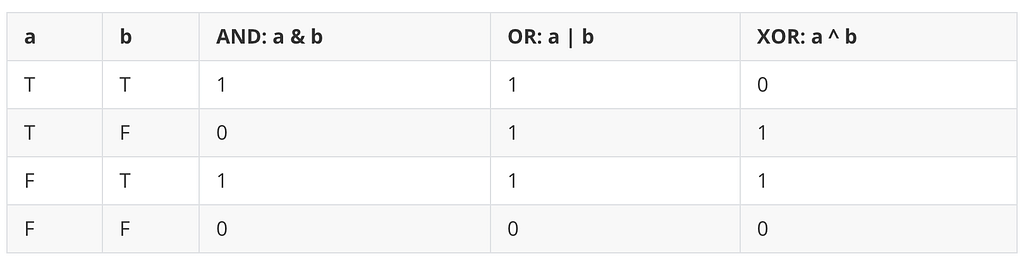The truth table for AND, OR, and XOR.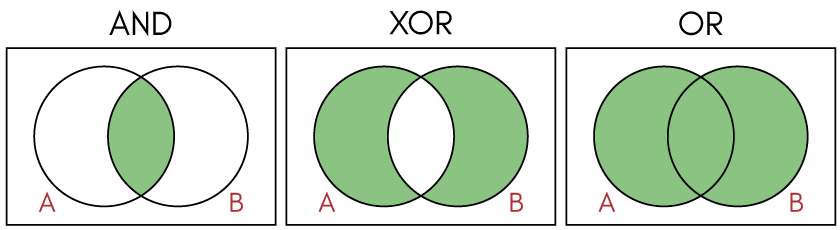AND, XOR, OR Venn diagrams

When you use XOR with even numbers of the same number, the output is always 0.

In Rust, you can use {:#b} to print binary.

Output:

`0 ^ 0 = 0Binary: 0 ^ 0 = 0b01 ^ 1 = 0Binary: 1 ^ 1 = 0b02 ^ 2 = 0Binary: 2 ^ 2 = 0b03 ^ 5 ^ 3 ^ 5 = 0Binary: 3 ^ 5 ^ 3 ^ 5 = 0b01 ^ 1 ^ 1 = 1Binary: 1 ^ 1 ^ 1 = 0b11 ^ 1 ^ 5 = 5Binary: 1 ^ 1 ^ 5 = 0b101`

You can find Python code here.

### Problem 1: Single Number

We are going to use this XOR to solve the LeetCoder problem called Single number.

In this problem, an array input has a pair of numbers except one, for example [1, 1, 5, 5, 2]. You need to find a sing number from this array and in this case the output should be 2.

More example: When the input is [2, 2, 1], the output should be 1. When an input is [4, 1, 2, 1, 2] the output should be 4.

This is a good example to use the XOR operator.

### Python Solution

We briefly go through the Python solution to see how the problem was solved.

Output:

`4`

Line 1: We use Python typing which is introduced from v3.5.

Line 3–4: After importing List, we create a class called Solution and method called singleNumber.

With Python type hints, we capitalize the name of the type, and set the name of the type inside the collection in brackets as seen above, num: List[int].

Line 5–8: We set a variable ans to 0. Using a for loop, we iterate the input array, nums using XOR compound assignment, ans ^= n. This will output the single number from the array.

Line 10–11: We instantiate the class Solution and call the method singleNumber.

(You can run this Python code without type notations if you are interested.)

The following is the solution for the LeetCode environment:

`class Solution:    def singleNumber(self, nums: List[int]) -> int:        ans = 0        for n in nums:            ans ^= n        return ans`

### Rust Code

Rust structs contain named fields. We use a keyword struct and set fields with its type within the curly bracket. We put methods into a impl block.

#### Starting code

Output:

`1`

Line 1: We suppress dead_code warning.

Line 2–4: Create a struct called Solution that takes one field nums with Vec<i32> type. (More on Vectors.)

Line 6–10: We create a method single_number in impl Solution. The single_number takes the first parameter &self (More on self .) and we just return 1 for now.

Line 12–17: In the main function, we create an instance and print 1 using the method.

It seems all working so we are going to complete the single_number method next.

### Method and Associated Functions

Methods are defined within the context of a struct and their first parameter is always self, which represents the instance of the struct the method is being called on. - The Rust Programming Language

Associated functions don’t take self as a parameter and they are not methods because they don’t have an instance of the struct to work with.

A good example is String::from function.

We use the :: syntax with the struct name to call this associated function whereas we use . when we call a method.

A common associated function is a new function that returns a value of the type the associated function is associated with.

Output:

`x: 5, y: 4x: 8, y: 9`

#### Final code

Line 7–11: We create a mutable variable ans with the type of i32 . Using for loop, we iterate &self.nums using ans ^=n.

Output:

`5`

We adjust the above code to the LeetCode environment.

`impl Solution {    pub fn single_number(nums: Vec<i32>) -> i32 {        let mut ans: i32 = 0;        for n in nums {            ans ^= n;        }        ans            }}`

The memory usage is 2.2 MB in Rust and 16.5 MB in Python. (More on Runtime & Memory usage)

### Solution Using an Associated Function

Since we learned about the associated function, let’s apply it to this problem.

Output:

`14`

Line 6–10: We create an associated function, new as we have done it before. This new function takes one parameter nums that is a vector with items of i32.

When the parameter names and the struct field names are exactly the same, we can use the field init shorthand syntax as nums instead of nums: nums.

In the main function, we call an associated function, new and pass nums as an argument. We use method syntax to call the single_number method on the ans3 instance.

### Problem 2: Number of Steps to Reduce a Number to Zero

In this problem, you input a non-negative integer num and return the number of steps to reduce it to zero. If the current number is even, you have to divide it by 2, otherwise, you have to subtract 1 from it.

For example:

`Input: num = 14Output: 6Explanation: Step 1) 14 is even; divide by 2 and obtain 7. Step 2) 7 is odd; subtract 1 and obtain 6.Step 3) 6 is even; divide by 2 and obtain 3. Step 4) 3 is odd; subtract 1 and obtain 2. Step 5) 2 is even; divide by 2 and obtain 1. Step 6) 1 is odd; subtract 1 and obtain 0.`
`Input: num = 8Output: 4Explanation: Step 1) 8 is even; divide by 2 and obtain 4. Step 2) 4 is even; divide by 2 and obtain 2. Step 3) 2 is even; divide by 2 and obtain 1. Step 4) 1 is odd; subtract 1 and obtain 0.`

This is a good example that we can use the Modulus/Remainder operator and the compound assignment operators.

#### Python Solution

Output:

`64`

Line: 3–10: We use a while loop for num > 0. If the modulus is 0, then it must be an even number so we divide the num by 2 using a compound assignment /=2, otherwise, we subtract 1 using a compound assignment -=1. We increase the steps by 1. Finally, we return the steps.

We adjust the above code to the LeetCode environment.

`class Solution:    def numberOfSteps (self, num: int) -> int:        steps = 0        while num > 0:            if num % 2 == 0:                num //= 2             else:                num -=1             steps += 1        return steps`A Python result from the LeetCode.

#### Rust Solution

Output:

`64`

In Rust, we take the same steps as we did in Python.

Line 7–16: We assign 0 to a mutable variable steps. While self.num is greater than 0, we use the compound assignment /=2 if self.num 's remainder is 0, otherwise, we subtract 1, and increase the number of step by 1.

We adjust the above code to the LeetCode environment.

`impl Solution {    pub fn number_of_steps (mut num: i32) -> i32 {        let mut steps = 0;        while num > 0 {            if num % 2 == 0 {                num /= 2;            } else {                num -=1;            }            steps += 1;        }        steps    }}`